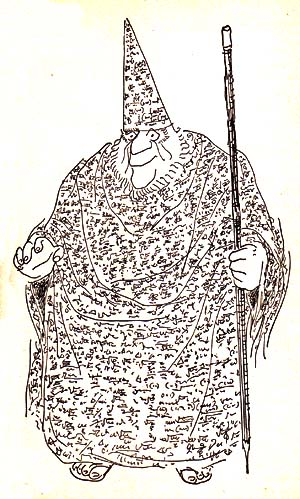# The Numbers Initials Puzzler

by TREVOR "The Games Man"

Instructions:  Each line contains the initials of words that will make a correct phrase.
Example:  12 = M in the Y.    Answer:  12 = Months in the Year.9 = P on a B T
9 = I in the G
9 = N in the Z P F
9 = P in the S S
9 = N in a S S N
76 = T in the O
1 = W (D N E)
64 = O in a G
2 = P in a Q
1492 = C S the O B
2, 4, 6, 8 = W D W A
2 = H M I T to T
50 = S on the F
4 = S in the Y
6 = S on a G
3 = S and Y O
12 = E in a D
13 = a B D
2 = C; 3 = a C; 4 = T M; 5 = N A
3 = L P
12 = S of I
12 = G in S
206 = B in the H S
50 = S in the U
50 = S in the W
50 = W to L Y L
64 = W Y S N M W Y S F M W I
23 = S
16 = S (and N B K)
18 = A of C
1, 2, 3, 5, 7, 13 = P N
1, 1, 2, 3, 5, 8, 13 = F S
30 = D H S A J and N
31 = H M A the R H (E F)
99 = B of B on the W
2 = S of R in P R B
150 = P in the B
96 = T (C C C)
7 = D S
100 = C in a D
5 = P in a N
144 = S I in a S F
16 = O in a P
366 = D in a L Y
92 = N E on E
40 = T with A B
80 = D to G A the W
1,000,000 = T A
2 = H M H I O G
100 = S in the U S S
64,000 = D the Q is W
1 = H M P I T T C a L B (B I R H T W T C)
100000000000000000000000000000000000000000000000000000000000000000
00000000000000000000000000000000000  = a G
4 = W T and T M
8 = L on a S
8 = T on an O
3 = on a M
99 = T O of a H
10 = G H
20,000 = L U the S
13 = O C
60 = S in a M
60 = M in an H
60 = S O T
52 = W in a Y
40 = H a W in a F T J
39 = J B A
6 = L on an I
13 = C in a S
500 = L at I
10 = I the B and the L O S R O
101 = D
8 = C in a V E E
2 = T F (and T F T)
2 = B B F
1, 2 = B M S
3, 4 = S T D
18 = W on a T T
66 = R Y G Y K O
20 = M in a B
4 = on the F
16 = T (W D Y G)
666 = the N of the B
500 = H of B C
1,000 = P of L
13 = W the C S W I T to G a N C
3 = C on my H
3 = D ( L, W, and H)
5 = S (S, S, H, T, and T)
7 = W of the W
1 = L, 2 = L, 3 = L I, 4 = L, 5 = L, 6 = L I, 7 = L, 8 = L, 9 = L I, 10 = L I B
151 = C L I
10 = Y for F D
360 = D in a C
4 = S in a D

for extra credit:
63 = T F N

TREVOR'S QUALITY GUARANTEE:
YOU WILL BE 100% SATISFIED AND
YOUR GROUP WILL HAVE A WHOLE LOT OF FUN...
OR IT'S FREE!

413-247-3322
trevor@trevorthegamesman.com
PO Box 463, Haydenville, MA 01039